# Electromagnetic Torques

The flux-surface integrated poloidal and toroidal electromagnetic torque densities acting on the plasma can be written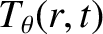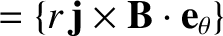(3.129)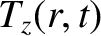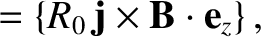(3.130)

respectively, where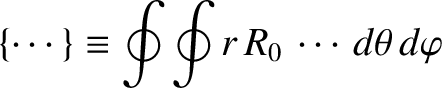(3.131)

is a flux-surface integration operator. However, according to the equations of marginally-stable ideal-MHD, (2.375)–(2.380), both the plasma equilibrium and the tearing perturbation satisfy the force balance criterion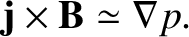(3.132)

Given that the scalar pressure is a single-valued function of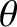and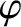, it immediately follows that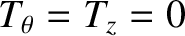throughout the plasma . The only exception to this rule occurs in the immediate vicinity of the rational surface, where Equation (3.132) breaks down. It follows that we can write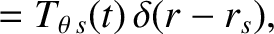(3.133)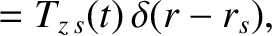(3.134)

where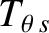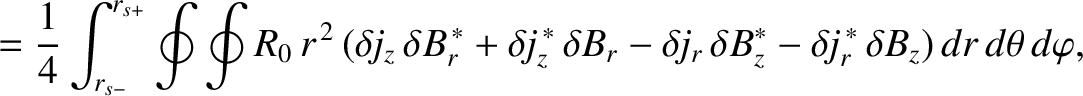(3.135)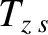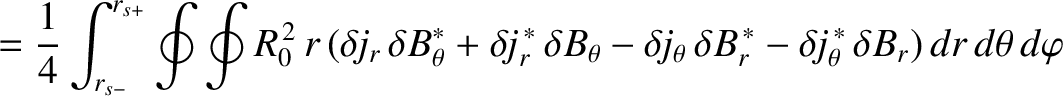(3.136)

are the net poloidal and toroidal torques, respectively, acting at the rational surface. Note that the zeroth-order (in perturbed quantities) torques are zero because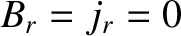. Furthermore, the linear (in perturbed quantities) torques average to zero over the flux-surface. Hence, the largest non-zero torques are quadratic in perturbed quantities.

Now, it follows from Equations (3.32)–(3.38) that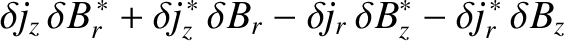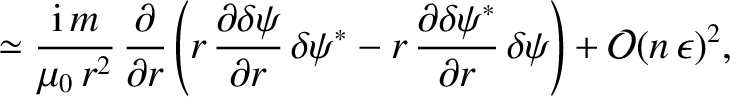(3.137)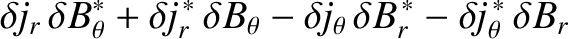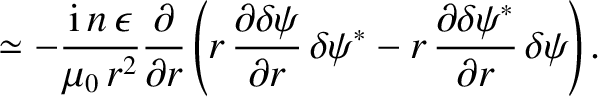(3.138)

The previous four equations yield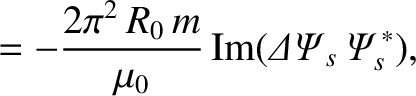(3.139)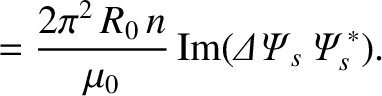(3.140)

where use has been made of Equations (3.72) and (3.73).

The net poloidal and toroidal electromagnetic torques acting on the resistive wall can be written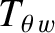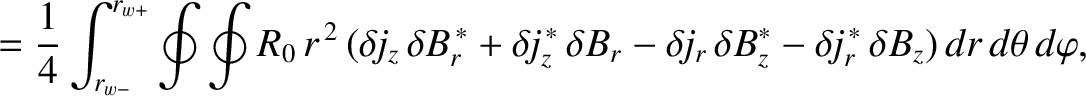(3.141)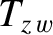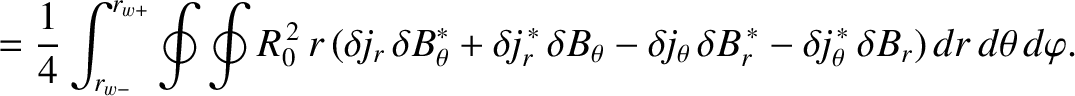(3.142)

Making use of Equations (3.32), (3.82), and (3.105)–(3.107), we obtain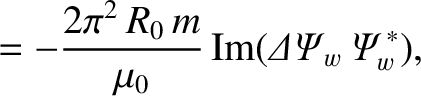(3.143)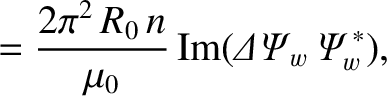(3.144)

The net poloidal and toroidal electromagnetic torques acting on the magnetic field-coil can be written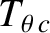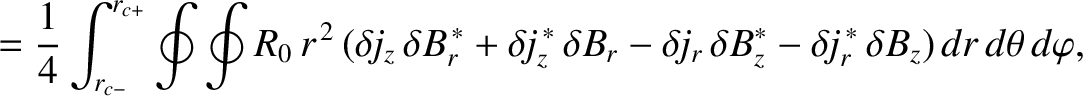(3.145)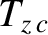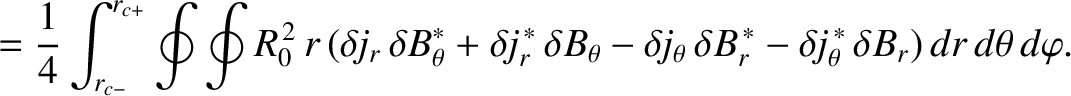(3.146)

Making use of Equations (3.32), (3.109)–(3.111), (3.120), and (3.122), we obtain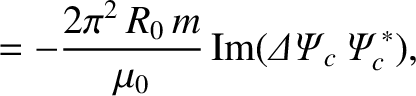(3.147)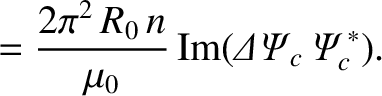(3.148)

It follows from Equations (3.123)–(3.125) that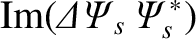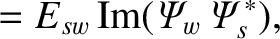(3.149)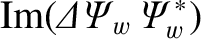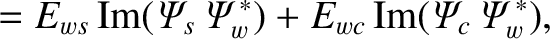(3.150)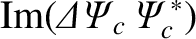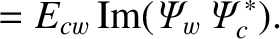(3.151)

Thus,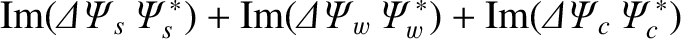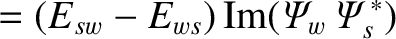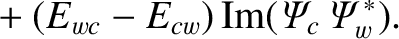(3.152)

However, according to Equations (3.90), (3.127), and (3.128),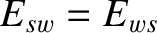and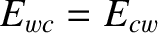. We deduce that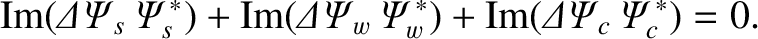(3.153)

Hence, Equations (3.139), (3.140), (3.143), (3.144), (3.147), and (3.148) yield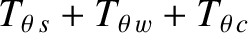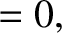(3.154)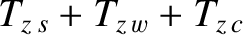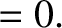(3.155)

In other words, the plasma/resistive wall/field-coil system exerts zero net poloidal electromagnetic torque, and zero net toroidal electromagnetic torque, on itself.